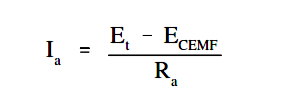# Generator Action in a Motor

A generator action is developed in every motor. When a conductor cuts lines of force, an EMF is induced in that conductor.

Current to start the armature turning will flow in the direction determined by the applied DC power source. After rotation starts, the conductor cuts lines of force. By applying the left-hand rule for generators, the EMF that is induced in the armature will produce a current in the opposite direction. The induced EMF, as a result of motor operation, is called counter-electromotive force, or CEMF, as illustrated in Figure 6.Since the CEMF is generated by the action of the armature cutting lines of force, the value of CEMF will depend on field strength and armature speed, as shown in Equation.

ECEMF = K Φ N

where

ECEMF = counter EMF
K = constant
Φ = field flux strength
N = speed of the armature

The CEMF opposes the applied voltage and functions to lower armature current. The effective voltage acting in the armature of a motor is the applied voltage, minus the counter EMF. Armature current can be found by using Ohm’s law, as shown in below Equation.where
Ia = armature current
ECEMF == terminal voltage
Et= counter EMF
Ra= armature resistance